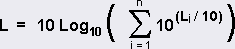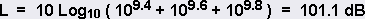USA ▼
Calculators > Decibel Calculator

# Decibel Calculator

The NoiseMeters decibel calculator can be used to carry out addition and subtraction of dB values between 0 and 200 dB.

## dB Calculator

0
dB